User Guide

# Lookups

Lookups are an important feature of Data Sync, these allow you to lookup values in other Data Sources much like a Foreign Key in a SQL Database. Data Sync is not limited to looking up against the same system type. For example your Source data might be Dynamics CRM and you can Lookup into a CSV file if you wish.

The Lookups create an In-Memory Table of all the values in the Lookup Data Source, this provides for a Fast Lookup without requiring a round-trip on every row to evaluate the lookup. Since the entire Data Source is loaded if the Lookup Data Set is large it can take a little while initially to create the Lookup Data Source.

## Create a Lookup

To create a Lookup first select the Column in the Data Source that you want to lookup values from. Then select the Add Lookup button from the mini-toolbar.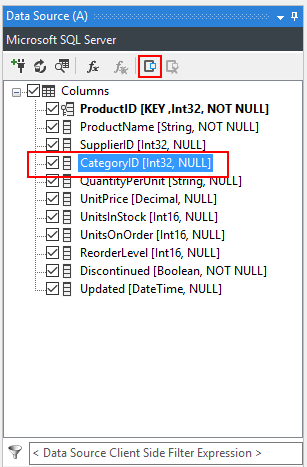You then create a connection to the target Data Source for the Lookup.

You can also simply drag and drop from the Connection Library onto the Column in the Data Source to create a Lookup.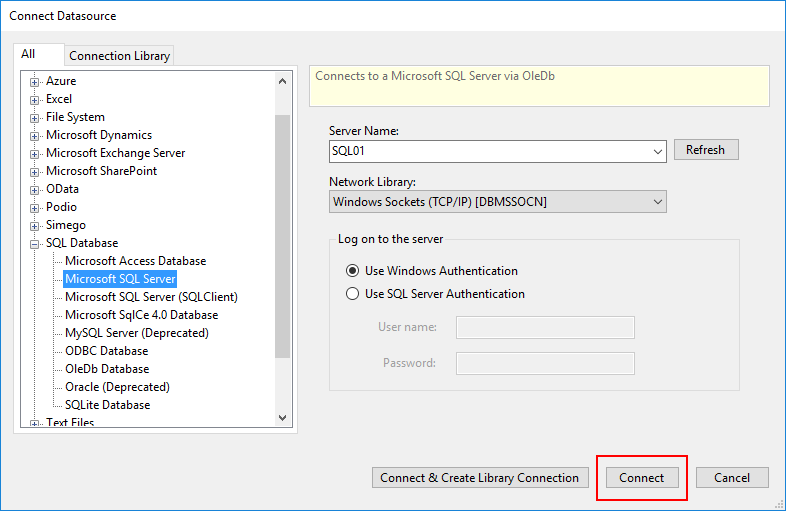Next you need to define the Lookup Connection properties, you should set the Data Type and the Column in the Target that will Join the Lookup Records.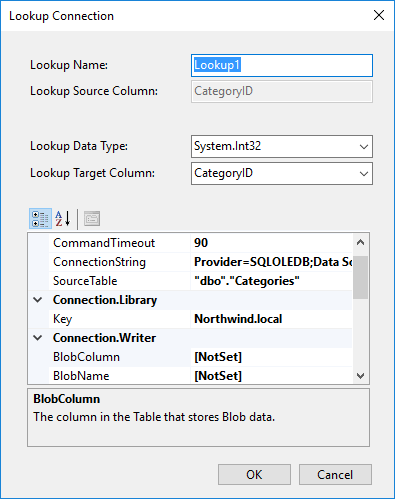Once the Lookup is configured you will notice a + against the column in the Data Source window with the Lookup Columns below. You can then use these columns just like any other column in your source.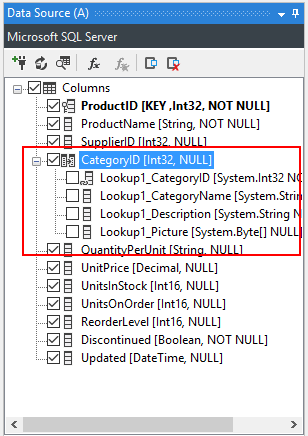## Delete a Lookup

To Delete a Lookup, select the Lookup and then press the Delete Lookup button from the mini-toolbar.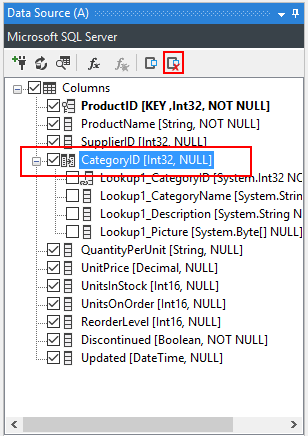## Calculated Column Lookups

The major Data Connectors support Lookups via Calculated Column Expression, these lookups re-use the connection on either the source or the target and are ideal when you need to get a value from your target system. These lookups create an internal dictionary of values that are used at runtime to return the values.

They are not the best option if you need many column values from the same lookup, in this scenario the normal lookups are better.

The functions LOOKUPA and LOOKUPB are used to return lookup data, LOOKUPA uses the Data Source (A) connection whilst LOOKUPB uses the Data Source (B) connection.

For example to lookup the value of the accountid in the Dynamics CRM account entity where the name field matches the Name column in your data source you would write the following expression.

``````LOOKUPB("accountid", "account", WHEN("name", Name))
``````

This is loosely equivalent to the following SQL

``````SELECT accountid FROM account WHERE name = ?
``````

You can also add additional WHEN parameters to the LOOKUPA/B function to create an AND expression.

i.e.

``````LOOKUPB("accountid", "account", WHEN("name", Name), WHEN("statuscode", MyStatusCode))
``````

This is loosely equivalent to the following SQL

``````SELECT accountid FROM account WHERE name = ? AND statuscode = ?
``````